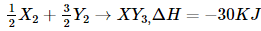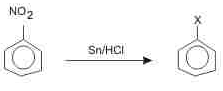JEE  >  WBJEE Chemistry Test - 11

# WBJEE Chemistry Test - 11

Test Description

## 40 Questions MCQ Test WBJEE Sample Papers, Section Wise & Full Mock Tests | WBJEE Chemistry Test - 11

WBJEE Chemistry Test - 11 for JEE 2022 is part of WBJEE Sample Papers, Section Wise & Full Mock Tests preparation. The WBJEE Chemistry Test - 11 questions and answers have been prepared according to the JEE exam syllabus.The WBJEE Chemistry Test - 11 MCQs are made for JEE 2022 Exam. Find important definitions, questions, notes, meanings, examples, exercises, MCQs and online tests for WBJEE Chemistry Test - 11 below.
Solutions of WBJEE Chemistry Test - 11 questions in English are available as part of our WBJEE Sample Papers, Section Wise & Full Mock Tests for JEE & WBJEE Chemistry Test - 11 solutions in Hindi for WBJEE Sample Papers, Section Wise & Full Mock Tests course. Download more important topics, notes, lectures and mock test series for JEE Exam by signing up for free. Attempt WBJEE Chemistry Test - 11 | 40 questions in 60 minutes | Mock test for JEE preparation | Free important questions MCQ to study WBJEE Sample Papers, Section Wise & Full Mock Tests for JEE Exam | Download free PDF with solutions
 1 Crore+ students have signed up on EduRev. Have you?
WBJEE Chemistry Test - 11 - Question 1

### Which one of the following species is capable of functioning both as Bronsted acid and Bronsted base ?

Detailed Solution for WBJEE Chemistry Test - 11 - Question 1

A Bronsted-Lowry acid is any species that is capable of donating a proton - H+
A Br insted-Lowry base is any species that is capable of accepting a proton, which requires a lone pair of electrons to bond to the H+
Water is amphoteric, which means it can act as both a Bronsted-Lowry acid and a Bronsted-Lowry base.
Strong acids and bases ionize completely in aqueous solution, while weak acids and bases ionize only partially.
The conjugate base of a Bronsted-Lowry acid is the species formed after an acid donates a proton. The conjugate acid of a Bronsted-Lowry base is the species formed after a base accepts a proton.
The two species in a conjugate acid-base pair have the same molecular formula except the acid has an extra H+ compared to the conjugate base.

WBJEE Chemistry Test - 11 - Question 2

### Phenol can be distinguished from ethyl alcohol by all reagents except

WBJEE Chemistry Test - 11 - Question 3

### Catalyst used in Rosenmund reduction is

WBJEE Chemistry Test - 11 - Question 4
The acidic solution of salt produced a deep blue colour with starch iodide solution. The salt may be
WBJEE Chemistry Test - 11 - Question 5

In Nessler's reagent, the ion present is

WBJEE Chemistry Test - 11 - Question 6
The spectrum of He is expected to be similar to that of
WBJEE Chemistry Test - 11 - Question 7

The four bonds in methane are directed in space at an angle of

WBJEE Chemistry Test - 11 - Question 8

Given that
C(s) + O₂(g) → CO₂(g); ∆H = - 395 KJ
S(s) + O₂(g) → SO₂(g); ∆H = -295 KJ
CS₂(l) + 3O₂(g) → CO₂(g) + 2SO₂(g); ∆H + -1110 KJ
The heat of formation of CS₂ is

WBJEE Chemistry Test - 11 - Question 9

The percentage of s-character in the hybrid orbitals sp, sp2 and sp3 follows the pattern

WBJEE Chemistry Test - 11 - Question 10
The correct statement in respect to protein haemoglobin is that is
WBJEE Chemistry Test - 11 - Question 11
According to Le-Chatelier's principle, adding heat to a solid and liquid in equilibrium will cause the
WBJEE Chemistry Test - 11 - Question 12
A foreign substance, that increases the rate of reaction, is called
WBJEE Chemistry Test - 11 - Question 13

A Carnot engine, working between 500 K to 300 K has a work output of 800 J-cycle-1. What is the amount of heat energy supplied to the engine from the source per cycle?

WBJEE Chemistry Test - 11 - Question 14
The IUPAC name of the following CH₃C(CH₃)₂CH₂CH=CH₂ is
WBJEE Chemistry Test - 11 - Question 15

Which one of the following cyano complexes would exhibit the lowest value of paramagnetic behaviour?

WBJEE Chemistry Test - 11 - Question 16
Paracetamol is/are
WBJEE Chemistry Test - 11 - Question 17
The substance not likely to contain CaCO₃ is
WBJEE Chemistry Test - 11 - Question 18
Molecular weight of a gas that diffuses twice as rapidly as the gas with molecular weigh 64 is
WBJEE Chemistry Test - 11 - Question 19
Which of the following is not a Lewis acid?
WBJEE Chemistry Test - 11 - Question 20
Mark the oxide which is amphoteric in character
WBJEE Chemistry Test - 11 - Question 21
Phosphorus normally exhibits a covalency of
WBJEE Chemistry Test - 11 - Question 22
The hydride H⁻ ion is a stronger base than OH⁻ ion.Which of the following reaction will occur if sodium hydride is dissolved in water?
WBJEE Chemistry Test - 11 - Question 23
Which of the following compounds will exhibit geometrical isomerism?
WBJEE Chemistry Test - 11 - Question 24

β- particles are

WBJEE Chemistry Test - 11 - Question 25
The major product (70%-80%) of reaction between m-dinitrobenzene with (NH4)2Sx is
Detailed Solution for WBJEE Chemistry Test - 11 - Question 25 (NH4)2Sx reduces one - NO2 to - NH2 group
WBJEE Chemistry Test - 11 - Question 26
Which of the following is plasticizer
WBJEE Chemistry Test - 11 - Question 27
WHich of the following polymer is an example of fibre?
WBJEE Chemistry Test - 11 - Question 28

How many Cl- ions are there around Na⁺ ion in NaCl crystal?

WBJEE Chemistry Test - 11 - Question 29
In haemoglobin the iron is in
WBJEE Chemistry Test - 11 - Question 30
Consider the following statements about enantiomers :
1. Isomers which are mirror images of each other are called enantiomers
2. A mixture of equal parts of enantiomers is called a racemic modification
3. Enantiomers show different properties-physical or chemical -only in a chiral medium
4. A racemic modification is converted by an optically active agent into mixture of diasteromers that can be separated.
Which of the above statements are correct ?
WBJEE Chemistry Test - 11 - Question 31

Aniline reacts with KOH and phosgene to form

WBJEE Chemistry Test - 11 - Question 32

Standard entropy of X₂,Y₂ and XY₃ are 60,40 and 50 JK-1 mol-1, respectively . For the reaction ,, to be equilibrium, the temperature will be

WBJEE Chemistry Test - 11 - Question 33In the above reaction X is

WBJEE Chemistry Test - 11 - Question 34

In the following question, a Statement of Assertion (A) is given followed by a corresponding Reason (R) just below it. Read the Statements carefully and mark the correct answer-
Assertion(A): The carbon-halogen bond in an aryl halide is shorter than the carbon-halogen bond in an alkyl halide.
Reason(R): A bond formed of an sp3 orbital should be shorter than the corresponding bond involving an sp2 orbital.

WBJEE Chemistry Test - 11 - Question 35

Toluic acid on reaction with Br₂ + Fe gives

WBJEE Chemistry Test - 11 - Question 36

WBJEE Chemistry Test - 11 - Question 37

Which one of the following conditions will increase the voltage of the cell represented by the following equation?
Zn(s) + Cu2+ (aq) → Zn2+ (aq) + Cu (s)

WBJEE Chemistry Test - 11 - Question 38

The sum of the number of neutrons and proton in the isotope of hydrogen is :

WBJEE Chemistry Test - 11 - Question 39

Which of the following will undergo aldol condensation ?

WBJEE Chemistry Test - 11 - Question 40

Which of the following compounds will react with ethanolic KCN ?

## WBJEE Sample Papers, Section Wise & Full Mock Tests

4 videos|10 docs|54 tests
 Use Code STAYHOME200 and get INR 200 additional OFF Use Coupon Code
Information about WBJEE Chemistry Test - 11 Page
In this test you can find the Exam questions for WBJEE Chemistry Test - 11 solved & explained in the simplest way possible. Besides giving Questions and answers for WBJEE Chemistry Test - 11, EduRev gives you an ample number of Online tests for practice

## WBJEE Sample Papers, Section Wise & Full Mock Tests

4 videos|10 docs|54 tests